Next: Standard rating formula Up: Details of the Rating Previous: Effective number of games

## Special rating formula

This procedure is to be used for players with either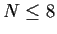, or players who have had either all wins or all losses in all previous rated games.

The algorithm described here extends the old provisional rating formula by ensuring that a rating does not decrease from wins or increase from losses. In effect, the algorithm finds the rating at which the attained score for the player equals the sum of expected scores, with expected scores following the provisional winning expectancy'' formula below. For most situations, the resulting rating will be identical to the old provisional rating formula. Instances that will result in different ratings are when certain opponents have ratings that are far from the player's initial rating. The computation to determine the special'' rating is iterative, and is implemented via a linear programming algorithm.

Define the provisional winning expectancy,''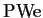, between a player rated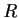and his/her-th opponent rated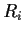to be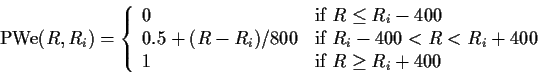Let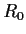be the prior'' rating of a player (either the pre-event rating for rated players, or the Step 1 imputed rating for unrated players), and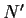be the effective number of games. Also let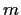be the number of games in the current event, and letbe the total score out of thegames (counting each win as 1, each loss as 0, and each draw as 0.5).

The variables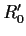and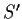, which are the adjusted initial rating and the adjusted score, respectively, are used in the special rating procedure. If a player has competed previously, and all the player's games were wins, then let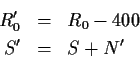If a player has competed previously, and all the player's games were losses, then let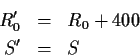Otherwise, let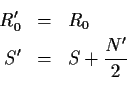The objective function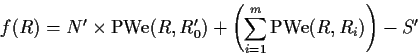which is the difference between the sum of provisional winning expectancies and the actual attained score when a player is rated, is equal to 0 at the appropriate rating. The goal, then, is to determine the value ofsuch thatwithin reasonable tolerance. The procedure to findis iterative, and is described as follows.

Let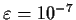be a tolerance to detect values different from zero. Also, let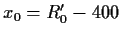,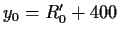, and, for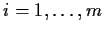,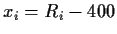,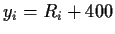. Denote the unique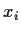and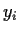,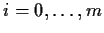, as the collection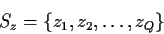If there are no duplicates, then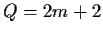. These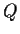values are the knots'' of the function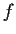(essentially the value where the function bends'' abruptly).

1. Calculate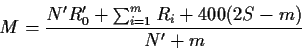This is the first estimate of the special rating (in the actual implemented rating program,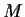is set to, but the final result will be the same - the current description results in a slightly more efficient algorithm).
2. If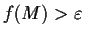, then
1. Let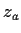be the largest value in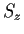for which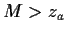.
2. If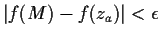, then set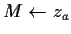. Otherwise, calculate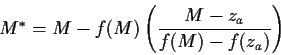• If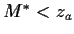, then set, and go back to 2.
• If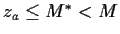, then set, and go back to 2.
3. If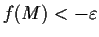, then
1. Let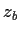be the smallest value infor which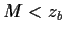.
2. If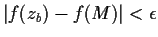, then set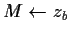. Otherwise, calculate• If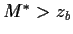, then set, and go back to 3.
• If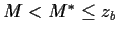, then set, and go back to 3.
4. If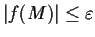, then let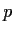be the number of,for which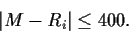Additionally, if, set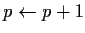.
1. If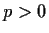, then exit.
2. If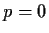, then letbe the largest value inandbe the smallest value infor which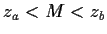. If
•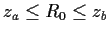, then set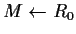.
•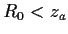, then set.
•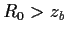, then set.
If the final value ofis greater than 2700, the value is changed to 2700. The resulting value ofis the rating produced by the special'' rating algorithm. Note that these computations do not depend on whether the event is a full-K'' or half-K'' event.Next: Standard rating formula Up: Details of the Rating Previous: Effective number of games
Mark Glickman
2004-09-22